# Lecture Power Points Chapter 17 Physics Principles with

• Slides: 26Lecture Power. Points Chapter 17 Physics: Principles with Applications, 6 th edition Giancoli © 2005 Pearson Prentice Hall This work is protected by United States copyright laws and is provided solely for the use of instructors in teaching their courses and assessing student learning. Dissemination or sale of any part of this work (including on the World Wide Web) will destroy the integrity of the work and is not permitted. The work and materials from it should never be made available to students except by instructors using the accompanying text in their classes. All recipients of this work are expected to abide by these restrictions and to honor the intended pedagogical purposes and the needs of other instructors who rely on these materials.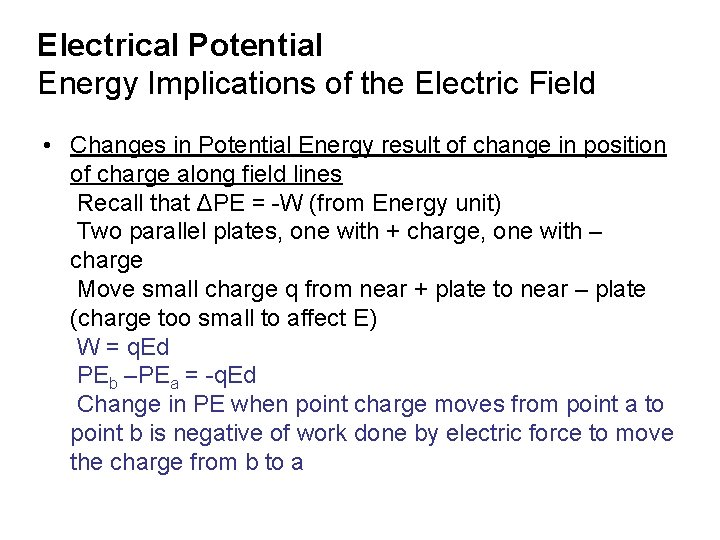Electrical Potential Energy Implications of the Electric Field • Changes in Potential Energy result of change in position of charge along field lines Recall that ΔPE = -W (from Energy unit) Two parallel plates, one with + charge, one with – charge Move small charge q from near + plate to near – plate (charge too small to affect E) W = q. Ed PEb –PEa = -q. Ed Change in PE when point charge moves from point a to point b is negative of work done by electric force to move the charge from b to a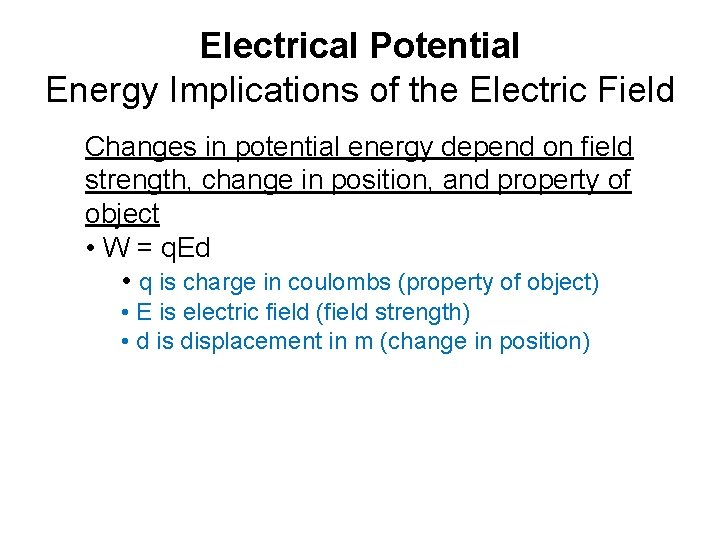Electrical Potential Energy Implications of the Electric Field Changes in potential energy depend on field strength, change in position, and property of object • W = q. Ed • q is charge in coulombs (property of object) • E is electric field (field strength) • d is displacement in m (change in position)Electrical Potential Energy Implications of the Electric Field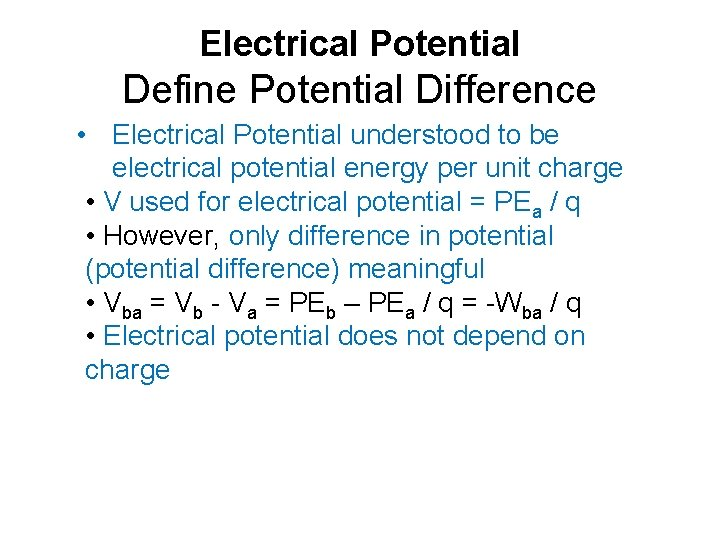Electrical Potential Define Potential Difference • Electrical Potential understood to be electrical potential energy per unit charge • V used for electrical potential = PEa / q • However, only difference in potential (potential difference) meaningful • Vba = Vb - Va = PEb – PEa / q = -Wba / q • Electrical potential does not depend on chargeElectrical Potential Define Potential DifferenceElectrical Potential Define Potential Difference • Positive plate at higher potential than negative (for positive test charge) • Positively charged object moves naturally from high potential to low potential • Negatively charged object does the reverse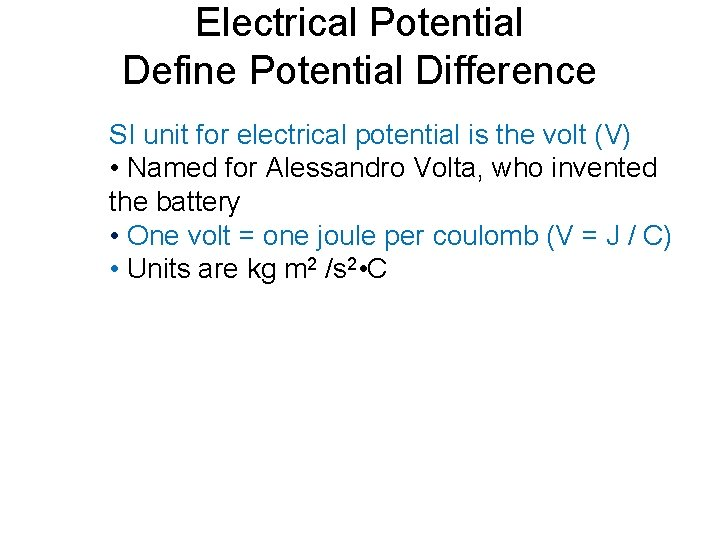Electrical Potential Define Potential Difference SI unit for electrical potential is the volt (V) • Named for Alessandro Volta, who invented the battery • One volt = one joule per coulomb (V = J / C) • Units are kg m 2 /s 2 • C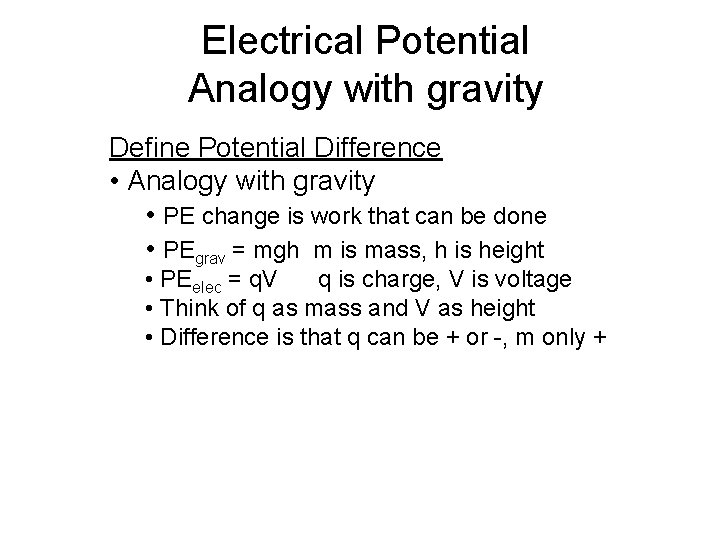Electrical Potential Analogy with gravity Define Potential Difference • Analogy with gravity • PE change is work that can be done • PEgrav = mgh m is mass, h is height • PEelec = q. V q is charge, V is voltage • Think of q as mass and V as height • Difference is that q can be + or -, m only +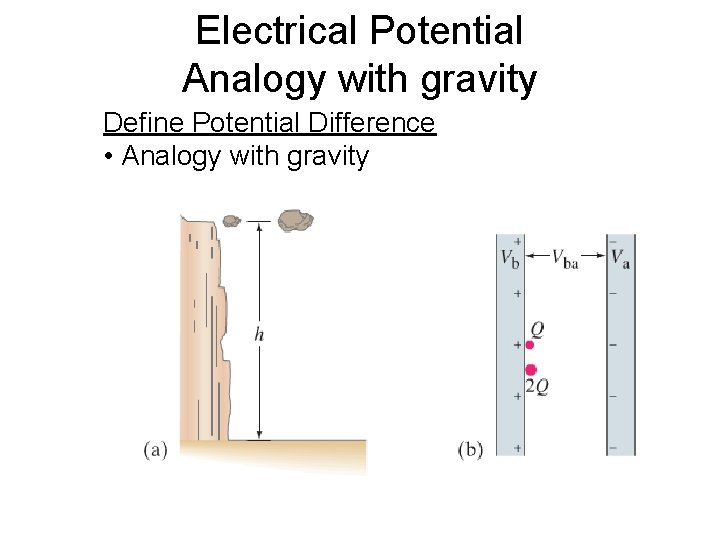Electrical Potential Analogy with gravity Define Potential Difference • Analogy with gravityElectrical PotentialElectrical Potential Electric Potential and Electric Field • Since W = -q. Vba • And W = Fd = q. Ed • Vba = -Ed • E = -Vba / d • - sign means electric field points in direction of decreasing potential V • So electric field can be written as volts / meter as well as newtons per coulomb • V/m=N/CElectrical Potential Map Equipotential Lines Equipotential lines/surfaces • Analogy with topographic mapElectrical Potential Map Equipotential Lines Equipotential lines/surfaces • Analogy with topographic map • On topographic map, contour lines are at same altitude • So contour lines are gravitationally equipotential • You can walk along a contour line and not change your PEgrav (not move up or down) • If you walk perpendicular to contour lines, you are changing PEgrav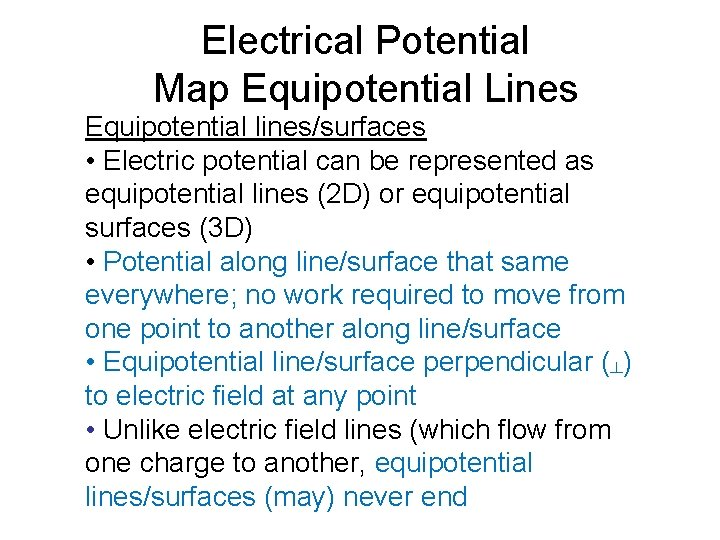Electrical Potential Map Equipotential Lines Equipotential lines/surfaces • Electric potential can be represented as equipotential lines (2 D) or equipotential surfaces (3 D) • Potential along line/surface that same everywhere; no work required to move from one point to another along line/surface • Equipotential line/surface perpendicular (┴) to electric field at any point • Unlike electric field lines (which flow from one charge to another, equipotential lines/surfaces (may) never end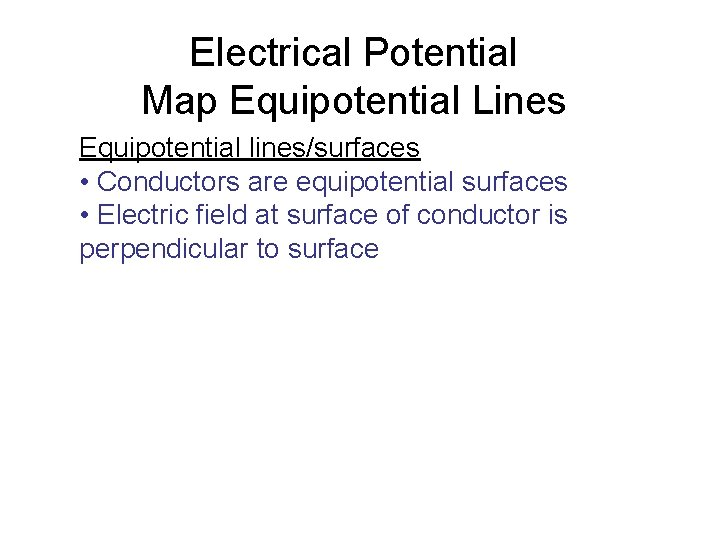Electrical Potential Map Equipotential Lines Equipotential lines/surfaces • Conductors are equipotential surfaces • Electric field at surface of conductor is perpendicular to surface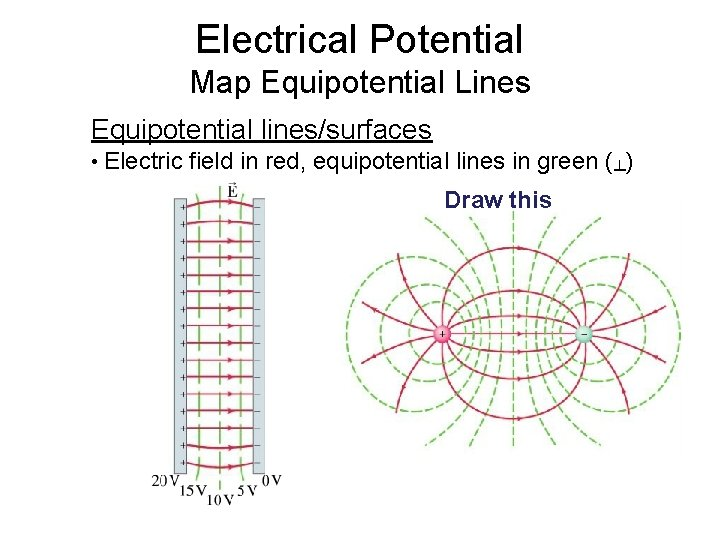Electrical Potential Map Equipotential Lines Equipotential lines/surfaces • Electric field in red, equipotential lines in green (┴) Draw thisEquipotential lines Potential difference: A to B = 100 V - 50 V = 50 V A to E = 100 V – (-100 V) = 200 V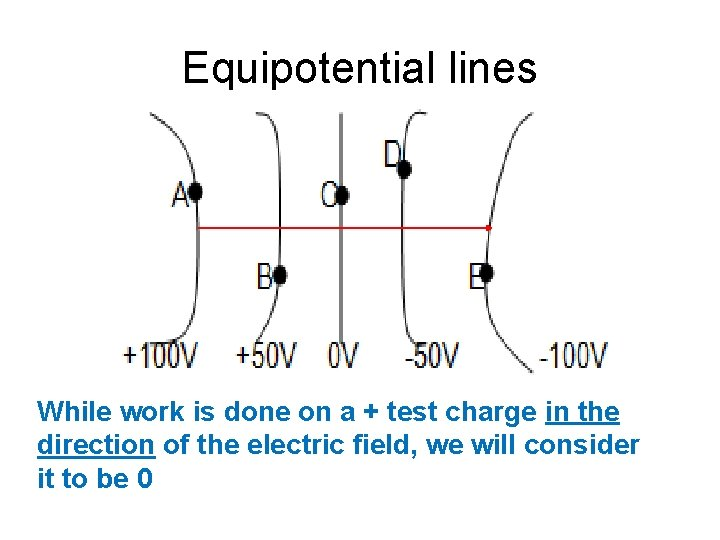Equipotential lines While work is done on a + test charge in the direction of the electric field, we will consider it to be 0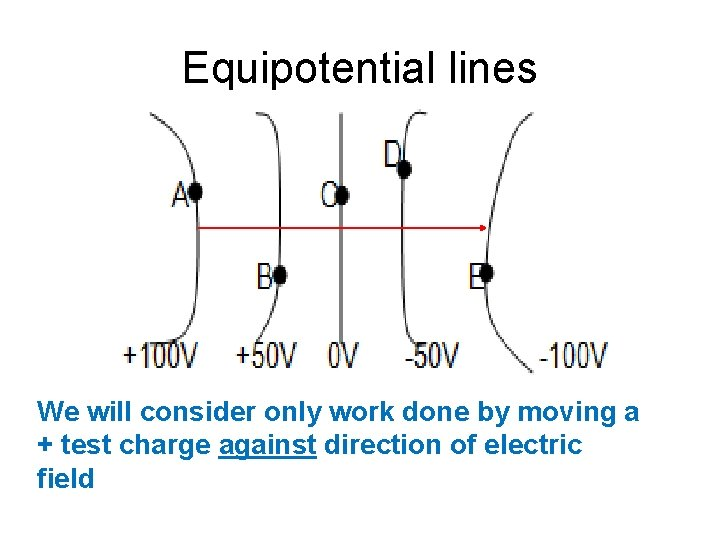Equipotential lines We will consider only work done by moving a + test charge against direction of electric field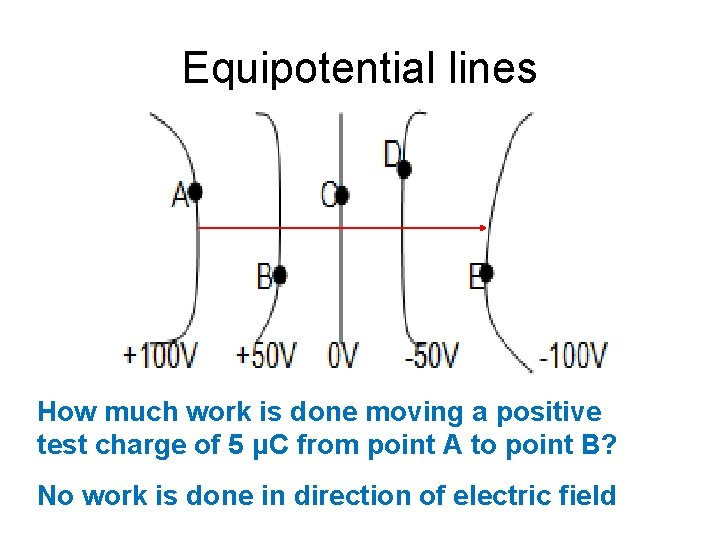Equipotential lines How much work is done moving a positive test charge of 5 μC from point A to point B? No work is done in direction of electric field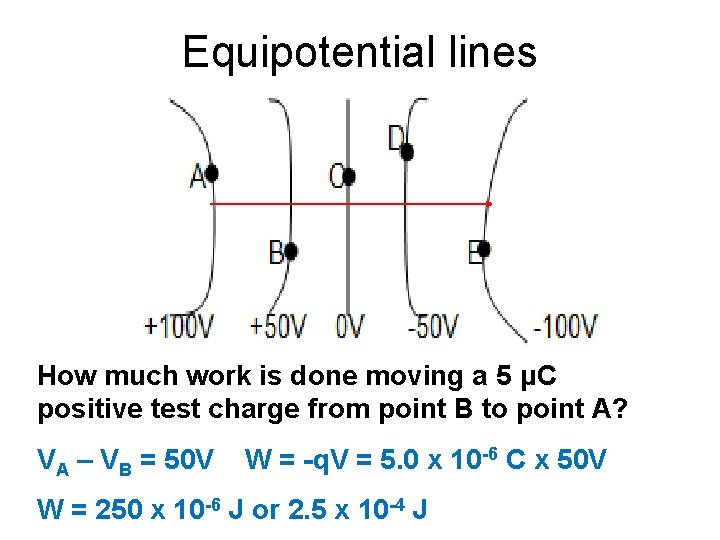Equipotential lines How much work is done moving a 5 μC positive test charge from point B to point A? VA – VB = 50 V W = -q. V = 5. 0 x 10 -6 C x 50 V W = 250 x 10 -6 J or 2. 5 x 10 -4 JApplications Electrocardiogram (EKG or ECG) is record of electric potential changes for given person’s heart Powerful tool in diagnosing heart defects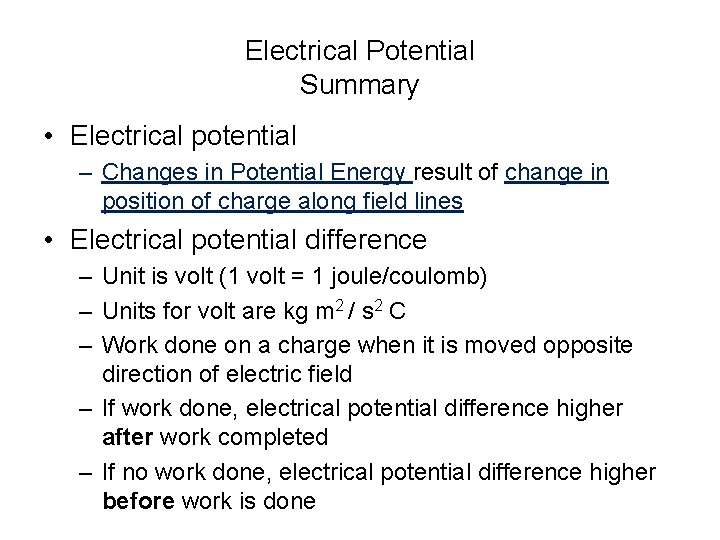Electrical Potential Summary • Electrical potential – Changes in Potential Energy result of change in position of charge along field lines • Electrical potential difference – Unit is volt (1 volt = 1 joule/coulomb) – Units for volt are kg m 2 / s 2 C – Work done on a charge when it is moved opposite direction of electric field – If work done, electrical potential difference higher after work completed – If no work done, electrical potential difference higher before work is done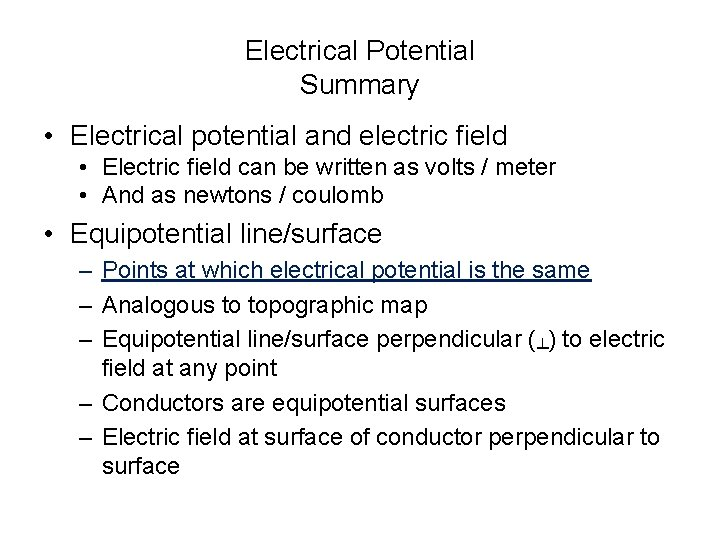Electrical Potential Summary • Electrical potential and electric field • Electric field can be written as volts / meter • And as newtons / coulomb • Equipotential line/surface – Points at which electrical potential is the same – Analogous to topographic map – Equipotential line/surface perpendicular (┴) to electric field at any point – Conductors are equipotential surfaces – Electric field at surface of conductor perpendicular to surfaceWater Analogy Source: http: //hyperphysics. phy-astr. gsu. edu/hbase/electric/watcir. html